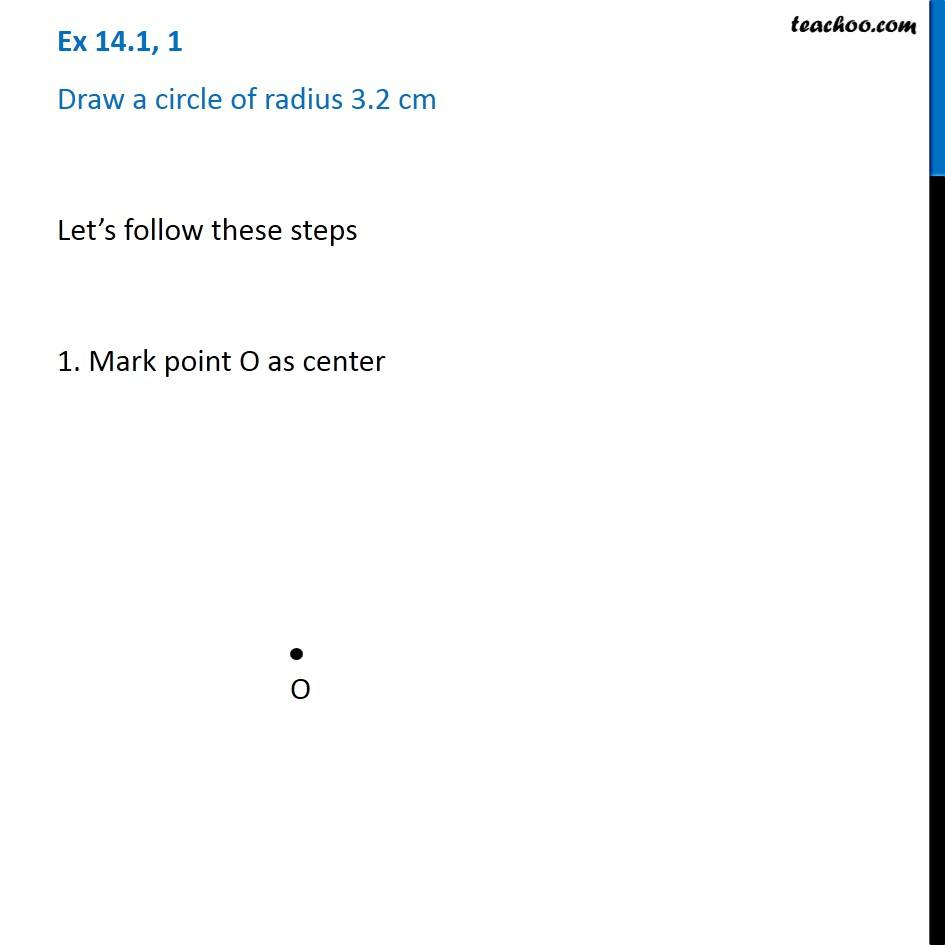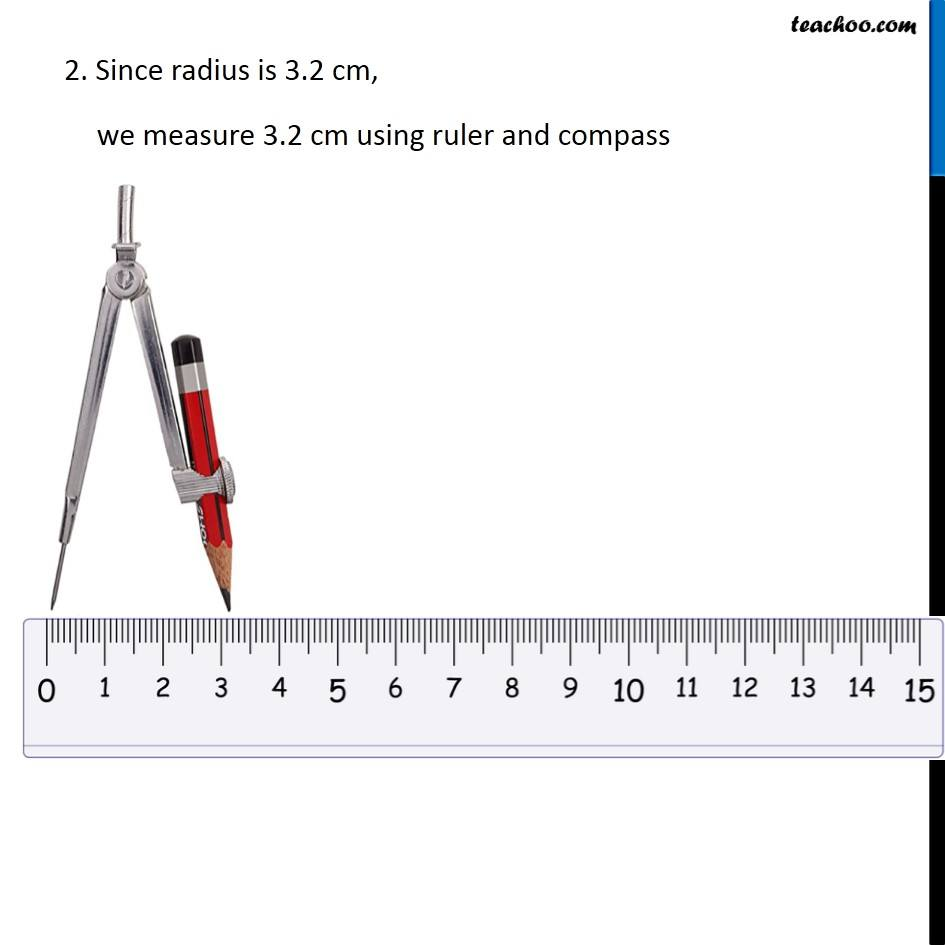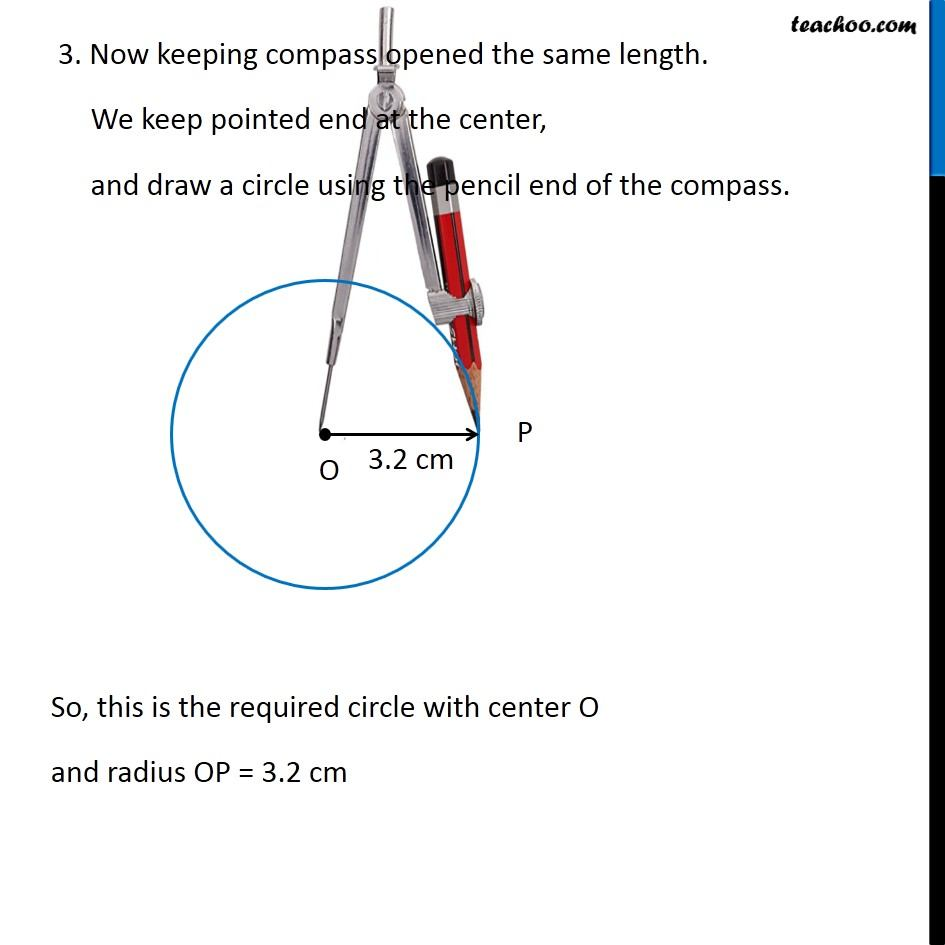Construction of a circle when its radius is known

Chapter 14 Class 6 Practical Geometry
Concept wise### Transcript

Ex 14.1, 1 Draw a circle of radius 3.2 cm Let’s follow these steps 1. Mark point O as center 2. Since radius is 3.2 cm, we measure 3.2 cm using ruler and compass 3. Now keeping compass opened the same length. We keep pointed end at the center, and draw a circle using the pencil end of the compass. So, this is the required circle with center O and radius OP = 3.2 cm